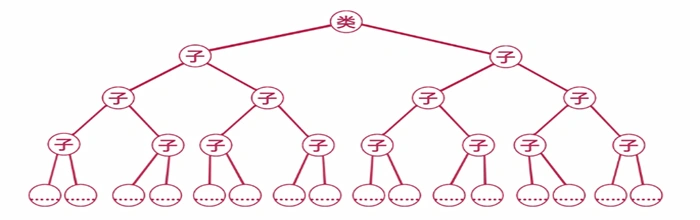# PHP使用递归算法查找子集获取无限极分类等实操``````/**
* 获取用户ID
*/
public function actionGetUserId()
{
\$model = new UserModel();
\$userInfo = \$model::find()->where(['level'=> 13])->asArray()->all();
\$userId = \$this->getInvId(\$userInfo,61);
var_dump(\$userId);
}``````

``````public function getInvId(\$data, \$invId)
{
static \$arr = [];
foreach (\$data as \$key => \$val) {
if (\$val['inv_id'] == \$invId) {
\$arr[] = \$val['id'];
\$this->getInvId(\$data, \$val['id']);
}
}
return \$arr;
}``````

## 3 条评论

•数据量很大会卡吗

•@Y 递归卡是正常的。。

•这个函数经常会用到的